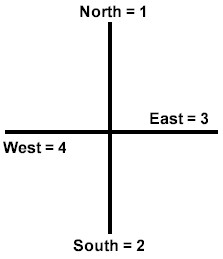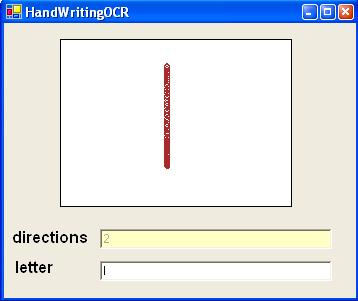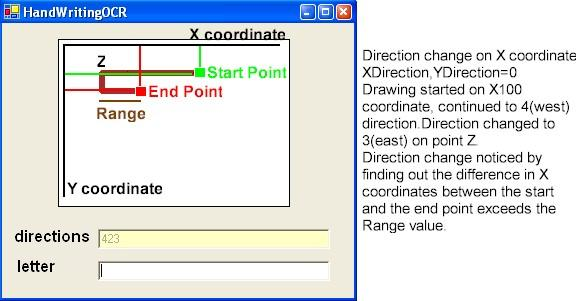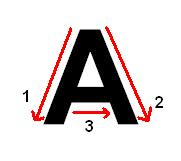# How to Interpret Handwriting with C#

In this article, I'll try to explain how to recognize handwriting with c#.

First of all, you might ask which projects we can use this kind of an app. At first shot you can use on public computers or palms etc. Of course there's a lot of components regarding this case at the market but the magic is to do it on your own using pure c#.

Let's figure out our algorithm and decide what type of controls we'll use in the project.

Algortihm:

User draws lines in different directions while he's writing. First we have to note this directions and give them a value.Direction Chart

We'll use a picturebox control so tht user can draw his handwriting on it.

Also we'll use two textboxes, one for showing the directions drawed instantly and one for showing the recognized letter.

Regarding the direction chart , the letter "I"'s direction signature will be only 2. If we simplify this, the user will draw a line to the south direction. We can achive the direction signature by storing the X and Y information when the user moves the mouse on the picturebox while pressing the left mouse button.

At this stage we have to check the direction changes so that we can build the direction signature.

Let's have a look at the following code.

private byte XDirection=0;
private byte YDirection=0;
private ArrayList X=new ArrayList();
private ArrayList Y=new ArrayList();
private StringBuilder OCR=new StringBuilder();
private byte History=10;
private int Range=5;
...
...
...
//Windows Form Designer generated code
...
...
private void pictureBox1_MouseMove(object sender, System.Windows.Forms.MouseEventArgs e)
{
bool XDirChanged=false;
bool YDirChanged=false;
if (e.Button==MouseButtons.Left)
{
Graphics g=pictureBox1.CreateGraphics();
g.DrawEllipse(
new Pen(Color.Brown), e.X ,e.Y,5,5);
if (X.Count>History)
{
if (XDirection==0)
{
if ((e.X-(int)X[X.Count-History])>Range)
{
XDirection=3;
XDirChanged=
true;
}
else if (((int)X[X.Count-History]-e.X)>Range)
{
XDirection=4;
XDirChanged=
true;
}
}
else
{
if ((e.X-(int)X[X.Count-History])>Range && XDirection==4)
{
XDirection=3;
XDirChanged=
true;
}
else if (((int)X[X.Count-History]-e.X)>Range && XDirection==3)
{
XDirection=4;
XDirChanged=
true;
}
}
}
if (Y.Count>History)
{
if (YDirection==0)
{
if ((e.Y-(int)Y[Y.Count-History])>Range)
{
YDirection=2;
YDirChanged=
true;
}
else if (((int)Y[Y.Count-History]-e.Y)>Range)
{
YDirection=1;
YDirChanged=
true;
}
}
else
{
if ((e.Y-(int)Y[Y.Count-History])>Range && YDirection==1)
{
YDirection=2;
YDirChanged=
true;
}
else if (((int)Y[Y.Count-History]-e.Y)>Range && YDirection==2)
{
YDirection=1;
YDirChanged=
true;
}
}
}
if (XDirChanged && XDirection!=0)
OCR.Append(XDirection);
if (YDirChanged && YDirection!=0)
OCR.Append(YDirection);
textBox1.Text=OCR.ToString();
}
}To do the drawing

We'll use the mousemove event of the picturebox to do the drawing. If the mouse is moving on the picturebox and the left mouse button is pressed we draw a shape to the X and Y location of the mouse.

...
if (e.Button==MouseButtons.Left)
{
Graphics g=pictureBox1.CreateGraphics();
g.DrawEllipse(
new Pen(Color.Brown), e.X ,e.Y,5,5);
...

To find out the direction changes

We have X and Y coordinates on a 2D environment. So the direction changes will occur either on X and Y coordinates. We'll use the XDirection and YDirection variables to find out the direction changes. If XDirection has a value of 3 the direction will be east, 4 will be west. If YDirection has a value of 1 the direction will be north, 2 will be south. If both these variables has a value of 0 then we'll understand that the user did not draw anything.

To find out the direction changes, it is the only way to match the current coordinate values with the previous ones. Thus we have to record the X,Y information drawed previously. To achieve this we'll use two ArrayList type variables named X and Y.

As we don't want our code to be that sensitive to record direction changes on one or two pixel changes, we'll use two variables named History and Range.

As you remember we'll track the direction changes by matching the previous x,y records with the current ones, History variable will keep the value that identifies how many records we'll return back in the arraylist. Range variable will keep the value of the maximum acceptable difference between the x,y values tht will be matched.

Let's have a look at the pictureHistory=10
Range=5

private void pictureBox1_MouseMove(object sender, System.Windows.Forms.MouseEventArgs e)
{
bool XDirChanged=false;
bool YDirChanged=false;
if (e.Button==MouseButtons.Left)
{
Graphics g=pictureBox1.CreateGraphics();
g.DrawEllipse(
new Pen(Color.Brown), e.X ,e.Y,5,5);
//checking if Arraylist variable X has enough members as history variable
if (X.Count>History)
{
//if the user is drawing for the first time
if (XDirection==0)
{
//if current X coordinate value is 5 pixels greater than the previous 10th record
//this indicates that the user is drawing through direction 3(east)
//We assign true to XDirChanged variable so the direction change will be stored in the arraylist
if ((e.X-(int)X[X.Count-History])>Range)
{
XDirection=3;
XDirChanged=
true;
}
//if previous 10th X coordinate value is 5 pixels greater than the current X value
//this indicates that the user is drawing through direction 4(west)
//We assign true to XDirChanged variable so the direction change will be stored in the arraylist
else if (((int)X[X.Count-History]-e.X)>Range)
{
XDirection=4;
XDirChanged=
true;
}
}
else
{
//if current X coordinate value is 5 pixels greater than the previous 10th record
//and the current direction is to the 4(west) this indicates that the user is
//drawing through direction 3(east)
//We assign true to XDirChanged variable so the direction change will be stored in the arraylist
if ((e.X-(int)X[X.Count-History])>Range && XDirection==4)
{
XDirection=3;
XDirChanged=
true;
}
//if previous 10th X coordinate value is 5 pixels greater than the current X value
//and the current direction is to the 3(east) this indicates that the user is
//drawing through direction 4(west)
//We assign true to XDirChanged variable so the direction change will be stored in the arraylist
else if (((int)X[X.Count-History]-e.X)>Range && XDirection==3)
{
XDirection=4;
XDirChanged=
true;
}
}
}
...

All these steps are the same for the Y coordinate.

To keep the direction changes on memory

We'll use a StringBuilder typed variable named OCR to achieve this. If the variable XDirChanged has a value of true and XDirection variable has a value different than 0 we add the direction to the OCR variable.
...
if (XDirChanged && XDirection!=0)
OCR.Append(XDirection);
if (YDirChanged && YDirection!=0)
OCR.Append(YDirection);
...

Final process, To interpret the direction changes

To start interpreting the direction changes user will have to click the right mouse button.By this event we'll interpret the changes by using AnaylseThis() method.

Before this grab a piece of paper and draw the letter A. Note the directions you draw.

(Remember the direction chart)

A letter
42323 ?

let's draw itWe go to direction 4 for X and 2 for Y as we draw line 1
We go to direction 3 for X and 2 for Y as we draw line 2
We go to direction 3 for X and 0 for Y as we draw line 3

Finally we can understand tht the 42323 value is letter A.

First thing you'll ask is tht the writing style may vary how we'll interpret different drawings for A?

Take a look at the following code.

private void AnalyseThis()
{
Graphics Gc=pictureBox1.CreateGraphics();
Gc.Clear(Color.White);
//Yorumlama;
string OCRText=OCR.ToString();
switch(OCRText)
{
//A
case "3123":
textBox2.Text+="A";
break;case "1323":
goto case "3123";
case "1332":
goto case "3123";
case "42233":
goto case "3123";
case "42323":
goto case "3123";
case "24323":
goto case "3123";
case "31323":
goto case "42233";
//B
case "2324324":
textBox2.Text+="B";
break;
case "231243124":
goto case "2324324";
case "232434":
goto case "2324324";
//C
case "423":
textBox2.Text+="C";
break;
case "243":
goto case "423";
//D
case "2324":
textBox2.Text+="D";
break;
case "2234":
goto case "2324";
//E
case "2333":
textBox2.Text+="E";
break;
//F
case "233":
textBox2.Text+="F";
break;
//G
case "42313":
textBox2.Text+="G";
break;
//H
case "223":
textBox2.Text+="H";
break;
case "232":
goto case "223";
//I
case "2":
textBox2.Text+="I";
break;
//J
case "241":
textBox2.Text+="J";
break;
//K
case "2423":
textBox2.Text+="K";
break;
case "2243":
goto case "2423";
//L
case "23":
textBox2.Text+="L";
break;
//M
case "22312":
textBox2.Text+="M";
break;
case "23212":
goto case "22312";
//N
case "2232":
textBox2.Text+="N";
break;
case "2322":
goto case "2232";
case "2321":
goto case "2232";
//O
case "42314":
textBox2.Text+="O";
break;
//P
case "22343":
textBox2.Text+="P";
break;
case "23243":
goto case "22343";
//R
case "223432":
textBox2.Text+="R";
break;
case "223423":
goto case "223432";
case "232432":
goto case "223432";
case "232423":
goto case "223432";
//S
case "4234":
textBox2.Text+="S";
break;
//T
case "32":
textBox2.Text+="T";
break;
//U
case "231":
textBox2.Text+="U";
break;
//V
case "2313":
textBox2.Text+="V";
break;
case "2331":
goto case "2313";
case "3213":
oto case "2313";
case "3231":
goto case "2313";
//Y
case "2312":
textBox2.Text+="Y";
break;
case "3212":
goto case "2312";
//Z
case "3423":
textBox2.Text+="Z";
break;
case "3243":
goto case "3423";
}
OCR.Remove(0,OCR.Length);
}

As you can see we can use different direction combinations for one letter. With this method we can interpret different handwriting styles.

This code can interpret wrongly for some similar letters like D,P. At this stage you can write some addins to solve this problem :)